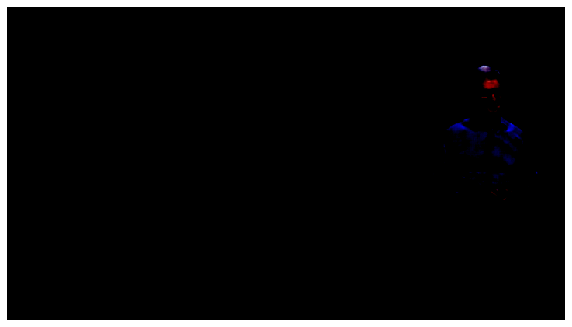# U-net predict/get_preds

Hello, I trained a u-net to remove jpeg artefacts from an image.
I exported the u-net to get the learner for inference.

learn.predict(IMG) works fine but I don’t want to use this function to predict one by one the images from a folder.

I tried to use learn.get_preds(ds_type=DatasetType.Test), but I don’t get why the predicted results are completely different from the results of the simple predict.

``````imgList = ImageImageList.from_folder(path / "Apple_vhs_test/", presort=True)

learn = load_learner("/models/", test=imgList [:1], tfm_y=False)

p = learn.get_preds(ds_type=DatasetType.Test)

Image(p).show(figsize=(10,10))
````````````fn = 'dataset/.../apple_VHS_0.jpg'
img_vhs = open_image(fn)

p,img_hr,b = learn.predict(img_vhs)
``````

Is there anything I can do to make `get_preds` behave as `predict`?